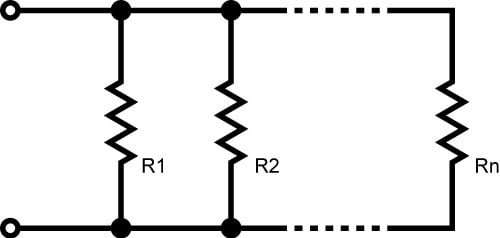# Parallel Resistance Calculator

## Calculate the total resistance of resistors in parallel with ease!

(‎Ω)
(‎Ω)

(Ω)

### How to Calculate the Total Resistance of Resistors in ParallelCalculating the equivalent resistance (REQ) of resistors in parallel by hand can be tiresome. This tool was designed to help you quickly calculate equivalent resistance, whether you have two or ten resistors in parallel. To use it, just specify how many parallel resistors there are and the resistance value for each one.

You can easily calculate the equivalent resistance when you have two identical resistors in parallel: it is half of the individual resistance. This is handy when you need a specific resistance value and don't have an appropriate part readily available. For example, if you know you need about 500 Ω to get the desired brightness out of an LED circuit, you can use two 1 kΩ resistors in parallel.

Keep in mind that the current through an individual resistor does not change when you add resistors in parallel, because adding resistors in parallel does not affect the voltage across the resistors' terminals. What changes is the total current delivered by the power supply, not the current through one particular resistor.

### Equations

$$\frac{1}{R_{EQ}} = \frac{1}{R_{1}} + \frac{1}{R_{2}} + \frac{1}{R_{3}} + ... + \frac{1}{R_{N}}$$

When you have only two resistors in parallel: $$R_{EQ} = \frac{R_1\times R_2}{R_1+R_2}$$

### Applications

Resistors in series are equivalent to one resistor whose resistance is the sum of each individual resistor. Resistors in parallel, on the other hand, result in an equivalent resistance that is always lower than every individual resistor. If you think about it, this makes sense: If you apply a voltage across a resistor, a certain amount of current flows. If you add another resistor in parallel with the first one, you have essentially opened up a new channel through which more current can flow. No matter how large the second resistor is, the total current flowing from the power supply will be at least slightly higher than the current through the single resistor. And if the total current is higher, the overall resistance must be lower.• M
mmikerosss September 15, 2017

Great tool, but there seems to be an error! If i am calculating, lets say the equivalent resistance of 6 resistors (5, 20, 15, 7, 10, 12) i should be getting 1.56ohms but instead i get 2.26ohms. Please try to fix the algorithm, otherwise great tool!

Like.
• R
Robert Keim September 21, 2017
I tried it with the same resistances and got 1.56 Ω, as expected. Are you still seeing 2.26 Ω?
Like.
•mrrobin November 15, 2017
I agree with you. I got same value.
Like.
•rwburbage April 13, 2019
And I agree you as well with 1.56. mmikerosss, you must have had a finger fault. So there, it's settled.
Like.
• Z
ZipZwan April 15, 2020
+1 for 1.56ohm result, so calculator is working as expected ;)
Like.
• J
jlhenager3 March 20, 2018

Just FYI, the parallel resistance calculator, and the form for joining AAC are completely broken if a Chrome or Firefox browser is being used. Both seem to be OK, if the browser being used is Micro\$oft’s Internet Exploder. Oh, the joy of making javascript work across multiple browsers!
Regardless, your tools are easy to use and handy.

Like.
• P
pll_check May 13, 2018

wrong calculator.. lol…
5,10,2,1 show 0.56, equivalent resistor is greater than 1.

Like.
•RK37 May 14, 2018
The calculator gives 0.56 Ω as the equivalent resistance of a 5 Ω, 10 Ω, 2 Ω, and 1 Ω resistor in parallel. This is the correct value.
Like.
•Anil Maharjan November 28, 2018
You have 1 ohm resistor. Adding other resistors in parallel will only decrease the resistance, so its not possible that the equivalent resistance is more than 1.
Like.
•Anil Maharjan November 28, 2018
You have 1 ohm resistor. Adding other resistors in parallel will only decrease the resistance, so its not possible that the equivalent resistance is more than 1.
Like.
• Q
Qute1 October 03, 2018

Doesn’t seem to work below 1ohm ? 3 x 0.01 gives 0.00 ???????

Like.
• C
C Mc February 20, 2020
The calculator does not go to 3 spots after the decimal and therefore, shows 0.00 0.01 / 3 = 0.00333--> 0.01 (resistor value) / 3 (number of resistors) = [parallel resistance value] With multiple resistors of the same value this is all you need to do to know the parallel resistance: Know the value of the resistor (R) Know how many resistors you are using (N) read the output (x) Here is the formula for total resistance of resistors of equal value when used in parallel: R / N = [x]
Like.
•thiodor Rodomagoulakis November 30, 2018

GREAT JOB GUYS!
KEEP ON THE GOOD WORK!

Like.
•Robson Robson July 31, 2019

Hello, It would be nice to add a button to confirm the number of resistors in parallel, because if you enter a high value, the page may freeze. Thank you.

Like.
• J